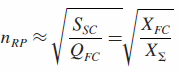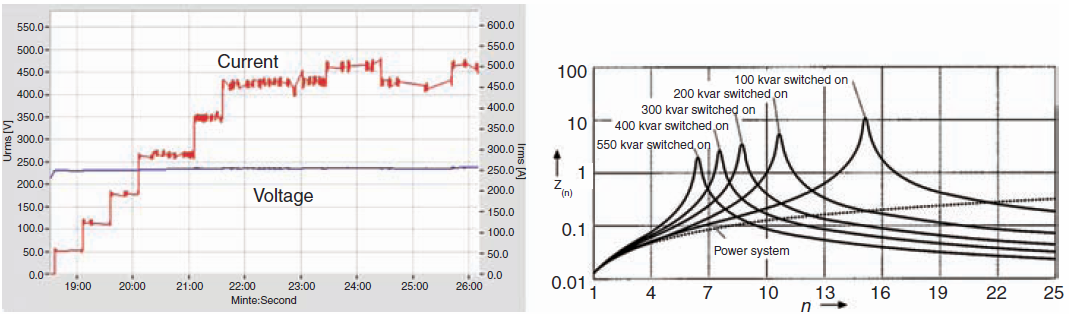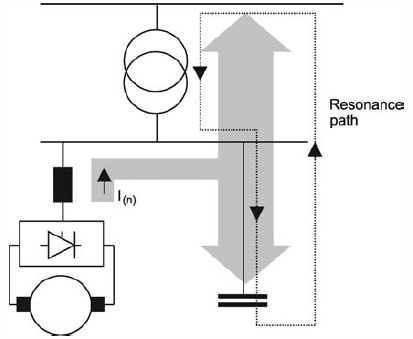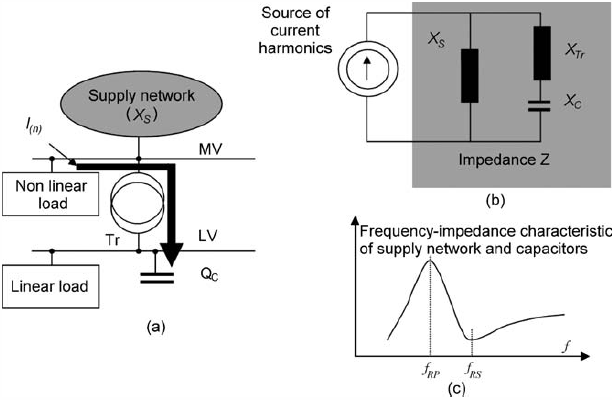# Series and Parallel Resonance

Author: Zbigniew Hanzelka

Source: Handbook of Power Quality Edited by Angelo Baggin, John Wiley & Sons, Ltd

## 1. PARALLEL RESONANCE

The power QFC of a capacitor bank intended exclusively for power factor correction should be selected with accuracy and care, taking into account the frequency–impedance character­istics at PCC (Figure 1). The problems which may result from the resonance phenomena, due to the presence of high harmonics, should be avoided. It should be ensured that the resonance frequency nRP of the circuit of the supply network–capacitor bank guarantees the circuit detuning from close to parallel resonance conditions, i.e. the resonance frequency is not close to any significant harmonic generated by the non-linear load.Figure 1 shows example frequency characteristics of the supply network and capac­itor bank, as seen from the non-linear load terminals. The increase of resonant frequency with the increase of the battery power (number of connected sections, capacitance) can be clearly seen.

Figure 2 shows a schematic diagram for the circuit where, for the effect of resonance phenomena, the current magnification occurs for one of the characteristic harmonics of a six-pulse converter drive.Figure 1 The current of a capacitor bank divided into sections and the frequency characteristics of the impedance of the supply network–capacitor bank, as seen from the non-linear load terminalsFigure 2 Amplification path of the harmonic current in the supply network–capacitor bank circuit

Detuning from parallel resonance can be achieved by selecting appropriate parameters of the compensation circuit, i.e. changing the battery power and/or adding an anti-resonance reactor. It is connected in series with the capacitor bank when voltage distortion is contained within the limits but the battery should be protected against overload due to excessive harmonic currents. The capacitor bank with a detuning reactor differs from a single-branch harmonic filter only in the series resonance frequency and its purpose. It should be emphasized that the bank with a detuning reactor also reduces the voltage distortion at busbars, although to a lesser extent than the filter does.

Connecting the reactor in series with the capacitor bank entails some additional require­ments for such an installation, namely:

• capacitor units should be rated for a voltage higher than the supply network nominal voltage (UC = Un2/(n2 −1), where n is the order of the component to which the LC series circuit is tuned;
• the reactors’ longitudinal insulation should be sized to withstand overvoltages caused by the bank switching currents. Overvoltage values depend on the LC parameters of the compensation circuit.

The selection of the resonant frequency of the series LC branch is of particular importance. It should be lower than the lowest harmonic frequency at the point of battery connection, so that the LC branch will have (for this frequency) an inductive character.

The series reactor is often selected using the so-called damping factor (p) defined as a quotient of the reactor voltage by capacitor voltage, expressed as a percentage. A damping factor of p = 5.67 % means the LC branch series resonance frequency is 223 Hz. Such a reactor is applied in networks where the fifth harmonic is dominant. Similarly for p = 7% (189 Hz) and 14 % (133 Hz).

## 2. SERIES RESONANCE

The series resonance may occur under certain conditions in a power system with capacitor banks installed. This case is illustrated in Figure 3. Low-voltage busbars are supplied from the distribution network through the transformer (Tr). Capacitor banks were connected for the purpose of reactive power compensation. A six-pulse thyristor converter (a non­linear load) is supplied from medium-voltage busbars. The equivalent circuit diagram and the frequency–impedance characteristic, seen from the converter terminals, are shown in Figure 3(b) and (c).Figure 3 (a) The schematic diagram, (b) the equivalent circuit, and (c) the frequency–impedance characteristics for an example installation with non-linear load and capacitor bank

It is evident that, apart from the parallel resonance for the frequency fRP, also the series resonance occurs in this circuit for the frequency fRS for which the total impedance attains a small value. This means that for this harmonic the resonant circuit exhibits the properties of a parallel filter. If the resonant frequency fRS is close to the fifth or seventh harmonic, for example, then this harmonic current, generated by the converter, will flow in the circuit of the transformer and the capacitor reactances connected in series. In this case, conversely to the parallel resonance, the harmonic current magnification does not occur, but the resonant circuit forces the current to flow in the circuit not intended for such a purpose. A strong voltage distortion may occur in the low-voltage circuit.